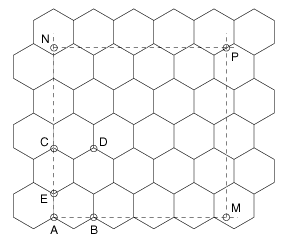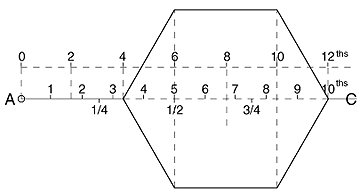# The Size of Brood-Comb Cells

The Size of Brood-Comb Cells

# The Size of Brood-Comb Cells

The Bee World – September, 1938 – Pages 106-107

By ANNE BETTS.

Many beekeepers who have been interested in the work of Baudoux and others on the influence of the brood-comb cells on the size and efficiency of bees bred in them, must have wished that it was easier to estimate the number of cells per square inch or square dm. of comb – without, that is, an elaborate calculation or the use of a graph (particularly as few of the latter have been published, and none, we believe, in English. See THE BEE WORLD, XVI, p. 82, for data from which a graph can be drawn).

This difficulty has also, it seems, been felt in Switzerland, and Herr M. Justrich, of the race-breeding organisation, takes up the subject in the August Schweizerische Bienenzeitung. He points out the technical difficulties which beset the measurement of the width of a cell and suggests the very happy idea that, instead of measuring the width, say, of 10 cells, we should count how many cells go into 10 cm. (1 decimetre). This number, multiplied by itself and again by 2.309, gives with very fair accuracy the number of cells on the two sides of a square decimetre of comb, which is the number generally used to express the size of cells. He gives a table, ranging from 12.8 to 20 cells per decimetre and (correspondingly) from 378 to 923 cells per sq. dm.

Now it is undoubtedly easier to most people to multiply three figures together than to multiply two together and then divide the product into a third. But even the easier process will call for a large piece of paper and perhaps a good deal of head-scratching on the part of beekeepers whose school days are some way behind them, and who do not habitually do any calculations other than the keeping of simple accounts.We therefore meditated a little on Herr Justrich’s idea, and are glad to report that it has led to a method which does away with the use of large numbers in multiplication. These large numbers are the result of the fact that the area of a six-sided cell, expressed in terms of one of its sides or of its width, inevitably contains the square root of 3 as a factor. This quantity is incommensurable – that is, the decimal expressing it never comes to an end – but the first few places are: 1.7320508. Hence we must take at least 1.732 when calculating with it, to get a sufficiently accurate result. It is possible, however, to get rid of this troublesome four-figure number by using the method which follows.

Place the comb on the table in front of you so that it is the same way up as in the hive, with the cells arranged “right way up.” Choose one of the upright sides of a cell and start from its bottom end (marked A in the diagram, and surrounded with a small circle to distinguish it). Measure a distance (10 cm. or one decimetre) across the comb, parallel to the top bar; and count how many cells there are in this length. (In the diagram, the cells are drawn much too large, especially if we imagine the diagram to be enlarged, so that A M really is 10 cm. and not about 6.5 cm., as it has been drawn; but the principle is of course the same whatever the size of the cells). In our diagram there are about 4.3 cells in A M.

Now consider the vertical line A C, consisting of the side at A and the whole height of the cell in the next row above. For shortness, we shall call this line a “height.” Measure A N vertically upwards, and of the the same length as A M (in practice, therefore, 10 cm.), and count how many “heights” like A C go into A N. In the case we have drawn there are about 2.4 of them.

Now consider the space A C D B. The portion just below C D is the same as the portion (outside A C D B) below A B; and it is thus easy to see that A C D B is equal to the area of two cells.

Our measurements and counts have therefore shown us that there are, in 10 X 10 cm., or 1 sq. dm., 4.3 X 2.4 areas like A C D B. Each of these areas is two cells, and there are also two cells on the other side of it; so that the number of cells per sq. dm. at once comes out as 4 X 4.3 X 2.4, or about 41, in this particular “comb.”

In an actual case of comb built on foundation, we found A M to contain 17.9 cells, while A N contained 10.7 “heights,” nearly. The number of cells per sq. dm. was, therefore, 4 X 17.9 X 10.7, or 766 cells (to the nearest whole cell).

If the bees’ cells were always perfect hexagons, A C would be exactly three times A E (the side of a cell), and A B would be A E X the square root of 3, or A E X 1,732 nearly. But in practice comb is seldom regular, particularly when built on foundation made in a roller-mill, so that it is better to count both A M and A N, and not to depend on the theory that the number of cells in A M must be 1.732 the number of “heights” in A N (apart altogether from the trouble of dividing by 1.732). For example, in the actual case above, there would have been only 10.3 “heights” in A N, had the comb been quite regular, and therefore about 737 cells per sq. dm, instead of 766.

Since it is not very easy to guess the fractions of A C, we have drawn a “height” on a large scale in Fig. 2, and marked on it the various fractions. It will be noticed that those who like to use the duodecimal system have an easy task, since the natural features of the comb make it very easy to see the 1/6th, 1/3rd, 1/2, etc., points.In most practical cases, one could take the nearest whole cell in each length. In the case mentioned, this gives 18 X 11 X 4, or 792 cells – an error of only 26 cells per sq. dm., or about 3.4%. The arithmetic can then often be done in one’s head.

Those who wish to use square inches instead of the metric system can easily do so. Take 2″ for the lengths A M, A N. Then A N P M will be 4 square inches, and it is only necessary to multiply together the figures obtained by counting, in order to get the cells per square inch. In the case of actual comb quoted 5.5 X 9.1, or 50 cells per square inch. (Since there are 15.5 square inches in one square decimetre, this gives 775 cells per square decimetre – a little too big, due partly to the estimate being rougher with a smaller length, and partly (perhaps) to variation in the cells in the different parts of the comb we measured. If it is desired to get the same accuracy as with the decimetre estimates, 4″ (which is nearly 10.2 cm.) should be used; but we must then divide the result by 4.

The truth is, comb varies a good deal; so that, for practical work – for example, when wishing to breed from the colony which builds the largest cells – the rough method (taking the nearest whole cell or “height” in each case) is probably good enough.

The making of a table connecting cells per decimetre (along A M) and number of cells per square decimetre is very easy, if we can assume (as Herr Justrich has done) that the cells are regular hexagons. We have only to take the number of cells in 1 dm., square it, divide it by the square root of 3 and multiply by 4 – a series of operations which can be done very fast on a slide rule and quite accurately enough to get good results. If readers would like such a table, we shall be pleased to publish one later on.